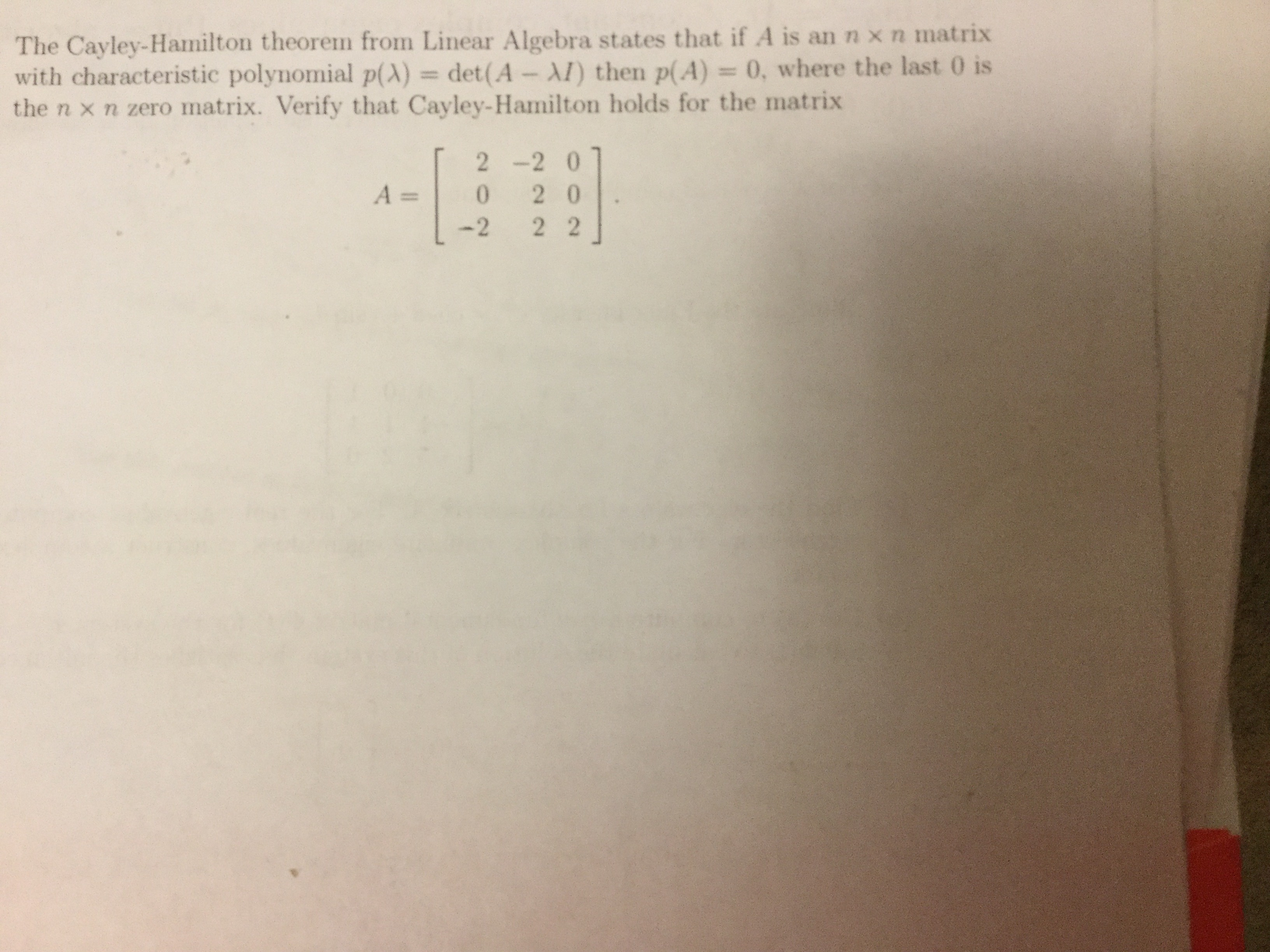# The Cayley-Hamilton theorem from Linear Algebra states that if A is an n x n matrix with characteristic polynomial p(λ-det(A-AI) then p(A) 0, where the last 0 is the n × n zero matrix. Verify that Cayley-Hamilton holds for the matrix 2 -2 0 A 0 2 0 -2 2 2

Question
290 views

Pls explain to me step by step
Thankshelp_outlineImage TranscriptioncloseThe Cayley-Hamilton theorem from Linear Algebra states that if A is an n x n matrix with characteristic polynomial p(λ-det(A-AI) then p(A) 0, where the last 0 is the n × n zero matrix. Verify that Cayley-Hamilton holds for the matrix 2 -2 0 A 0 2 0 -2 2 2 fullscreen

### Want to see the step-by-step answer?